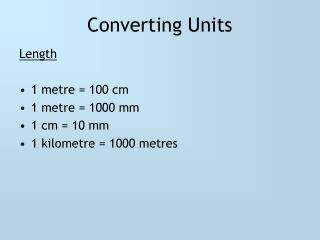DownloadDownload PresentationConverting Units

# Converting Units

Download Presentation## Converting Units

- - - - - - - - - - - - - - - - - - - - - - - - - - - E N D - - - - - - - - - - - - - - - - - - - - - - - - - - -
##### Presentation Transcript

1. Converting Units Length • 1 metre = 100 cm • 1 metre = 1000 mm • 1 cm = 10 mm • 1 kilometre = 1000 metres

2. Converting Units Weight • 1 kilogram = 1000 grams • 1 tonne = 1000 kilograms

3. Converting Units Volume • 1 litre = 1000 millilitres

4. Rules for Converting • If you are converting from a large to a small unit, then Multiply • If you are converting from a small to a large unit, then Divide

5. Questions

6. Questions